# Machine Learning Questions and Answers (Questions 31 to 40)

Welcome back! So we’ll continue with Questions 31 to 40 of our Machine Learning Q&A.

You can find Question 1 to 20 below

Questions 21 to 30

##### 31. Briefly Explain the Concept of Neural Network

Note that here, we are talking about Artificial Neural Network(ANN).

In simple terms, a neural network is a computing system made up of of interconnected nodes (called neurons) the that tries to model the behavior of biological (or animal) systems. It is normally represented as a directed graph.

Each neuron in a neural network receive a signal from its input, process it and then sent the output the the next neuron.

The neurons are connected by edges, each of which has a weight associated with it. The weight adjusts through a learning process.

Components of a neural network include:

an activation aj(t): this is the current state of the neuron

a threshold θj: a value such that when exceeded, the neuron produces a 1

an activation function : a function that computes new activation

an output function: a function that computes the output from the activation

##### 32. What is Feed-Forward Neural Network

A feedfoward neural network is a class of neural network where the connections between the neurons does not form a cycle. The information moves just in the forward direction, going from the input to the output through hidden nodes. They are considered simple.

Cycles or loos are not formed.

Examples of feedfoward networks are the perceptron and the multilayer perceptron.

##### 33. What is a Perceptron? What is Multilayer Perceptron?

As mentioned in question 32, the single-layer and multilayer perceptron are the simplest types of neural network.

Think of a perceptron as a neural network with a single neuron. It consists of set of inputs: x1, x2, . . . , xn and a function that maps its inputs x to an output y = f(x). The output of a perceptron could either be a 1 or a 0. This is given by: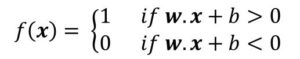where w is a vector of the weights of the inputs

w.x is the dot product of the weight and input such that: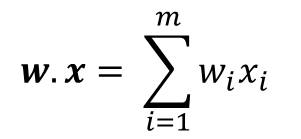m is the number of inputs

b is the bias

##### 34. What is a Sigmoidal Neuron

The sigmoid neuron is sometimes referred to as the building block of deep neural network. To understand the sigmoidal neuron, you first need to understand the sigmoid function. This is because a sigmoidal neuron is based on the sigmoid function.

A sigmoid function is  a mathematical function that produces the sigmoid curve (a curve that has the characteristic ‘S’ shape). An example is shown below: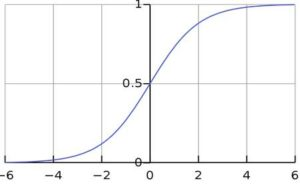The sigmoid neuron is similar to the perceptron except that for the sigmoid neuron, the output is a smooth curve while for the perceptron, we have a stepped function. An example of the sigmoid function is the logistic function which is given by: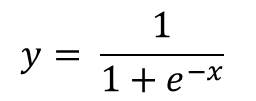Another example of a sigmoidal function is the hyperbolic tangent, tanh. This is given by the formula: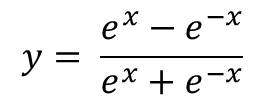##### 35. What is Network Parameter Optimization

This is the process of adjusting the network parameters in order to improve the performance of the network. On way is to adjust the weights of the edges in terms of the error they contributed.

During optimization, two phases are carried out:

• propagation
• weight update

propagation: when an input vector enters the input layer, it is propagated forward layer by layer through the network. When it gets to the output, then the output is compared to the correct output. The difference is an error given by a loss function E(w).

The error value is calculated for each neuron in the output layer. Then the errors are propagated backwards (backpropagation) through the network. For each neuron, the gradient of the loss function is calculated.

weight update: in this phase, the gradient calculated in the propagation phase is used. This gradient is then fed into the optimization method to update the weights of the neurons. the objective is to minimize the loss function.

##### 36. What is a Jacobian Matrix in Neural Network?

This is a matrix whose elements are given by the derivatives of the network output taken with respects to its inputs.

It is given by: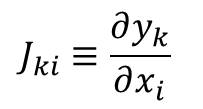where each derivative is computed for a particular input with all other inputs held constant.

Jacobian matrix helps to measure how sensitive the output is to changes in the inputs.

##### 37. What is Markov Chain?

Markov chain is a stochastic model (random or probabilistic model) used for modelling a sequence of possible events. It is such that he probability of each event depends on the state of the previous event.

Events in a Markov Chain must satisfy the Markov property: predictions on future events can be made based only on the present state.

##### 38. What is Irreducibility and Aperiodicity?

Irreducibility is a property of a Markov chain that states the we can reach any other state in a finite time irrespective of the present state.

Let’s take and axample of S = {s1, s2, s3, s4, s5}

The Figure below gives an example of irreducible and not irreducible Markov Chain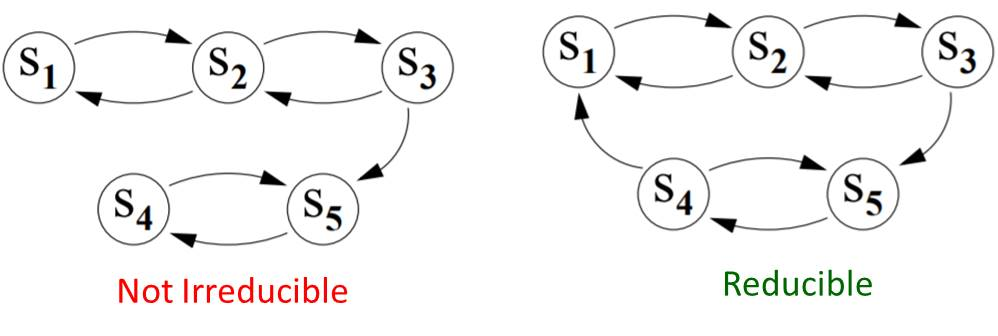Periodicity: This describes the period of occurrence that a state in the chain has. So if a state si in the chain has a period of 2, then the chain can be in state si every 2nd time depending on where we start.

It means it could be at even times or odd times but not both. If a state has a period of 1, then it is described as aperiodic

The figure above shows three chains where one has a period of 2 while others are aperiodic.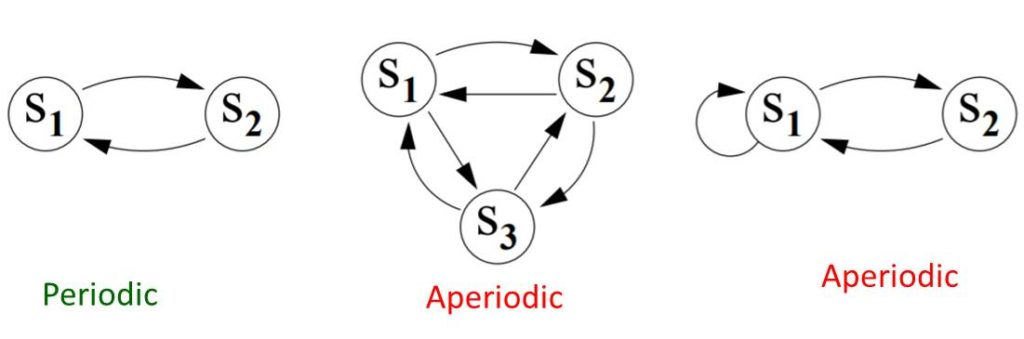##### 39. Explain the Metropolis-Hastings Sampling

This is related to question 38 on Markov Chain.

This is Markov-Chain-Monte-Carlo(MCMC) based sampling method where sequence of samples are obtained from a probability distribution where direct sampling may not be feasible.

Now, MCMC method is a technique for sampling from a probability distribution by constructing a Markov Chain with the required distribution.

##### 40. Explain the Concept of d-Separation in Probability

The concept of d-separation is related to dependence in probability. In fact the d- stands for dependence.

So two variables are considered to be d-separated relative to another set of variables Z in a directed graph if they are conditionally independent on Z on all the probability distributions that can be represented by the graph.#### kindsonthegenius

Kindson Munonye is currently completing his doctoral program in Software Engineering in Budapest University of Technology and Economics

View all posts by kindsonthegenius →

## One thought on “Machine Learning Questions and Answers (Questions 31 to 40)”

1.wooVar says:

hi, i am woo from Sweden and i want to explain any thing about “pandemic”. Please ask me 🙂